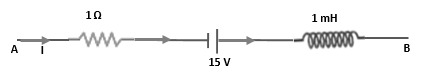The current through a choke coil increases from zero to 6 A in 0.3 seconds and an induced emf of 30 V is produced. The inductance of the coil is

1.  5 H

2.  2.5 H

3.  1.5 H

4.  2 H

Concept Questions :-

Self-inductance
High Yielding Test Series + Question Bank - NEET 2020

Difficulty Level:

A rod of length l rotates with uniform angular velocity $\mathrm{\omega }$ about an axis passing through its one end and perpendicular to its length. If a uniform magnetic field exists perpendicular to the axis of rotation, then induced emf across the two ends of the rod is :

1. $\frac{1}{2}B\omega {l}^{2}$

2. $B\omega {l}^{2}$

3.

4. zero

Concept Questions :-

Motional emf
High Yielding Test Series + Question Bank - NEET 2020

Difficulty Level:

Energy stored in an inductor can be made four times when

1. inductance is doubled

2. Current is made $\sqrt{2}$ times

3. current is doubled

4. can never to be made

Concept Questions :-

Self-inductance
High Yielding Test Series + Question Bank - NEET 2020

Difficulty Level:

A coil wire of a certain radius has 600 turns and a self-inductance of 36 mH. The self-inductance of a second similar coil of 500 turns will be

1.  25 mH

2.  50mH

3.  108mH

4.  75mH

Concept Questions :-

Self-inductance
High Yielding Test Series + Question Bank - NEET 2020

Difficulty Level:

The current passing through a choke coil of 5 H is decreasing at the rate of 3 As-1. The emf induced in the coil is

1. 15 V

2. -15 V

3. $\frac{5}{3}V$

4. $\frac{-5}{3}V$

Concept Questions :-

Self-inductance
High Yielding Test Series + Question Bank - NEET 2020

Difficulty Level:

If all linear dimensions of an inductor are tripled, then self-inductance will become (keeping the total number of turns per unit length constant)

1.  3 times

2.  9 times

3.  27 times

4. 1/3 times

Concept Questions :-

Self-inductance
High Yielding Test Series + Question Bank - NEET 2020

Difficulty Level:

A coil of resistance 20$\Omega$ and inductance 5H has been connected to a 200 V battery. The maximum energy stored in the coil is

(1) 250 J

(2) 125 J

(3) 500 J

(4) 100 J

Concept Questions :-

Self-inductance
High Yielding Test Series + Question Bank - NEET 2020

Difficulty Level:

A coil has, 1,000 turns and 500 $c{m}^{2}$ as its area. The plane of the coil is placed at right angles to a magnetic induction field of . The coil is rotated through 180$°$ in 0.2. The average e.m.f. induced in the coil, in milli-volts, is

(1) 5

(2) 10

(3) 15

(4) 20

Concept Questions :-

Faraday 's law and lenz law
High Yielding Test Series + Question Bank - NEET 2020

Difficulty Level:

The network shown in the figure is part of a complete circuit. If at a certain instant, the current I is 5 Amp and it is decreasing at a rate of $5×{10}^{3}$ A/s, then ${\mathrm{V}}_{\mathrm{B}}-{\mathrm{V}}_{\mathrm{A}}$ is equal to1. 20 V

2. 15 V

3. 10 V

4. 5 V

Concept Questions :-

LR circuit
High Yielding Test Series + Question Bank - NEET 2020

Difficulty Level:

A small square loop of wire of side l is placed inside a large square of wire of side L (L>>l). The loops are co-planar and their centres coincide. The mutual inductance of the system is proportional to :

1. l/L

2. ${\mathrm{l}}^{2}/\mathrm{L}$

3. L/l

4. ${\mathrm{L}}^{2}/\mathrm{l}$

Concept Questions :-

Mutual-inductance